## Water Images

#### Water Images

1. Choose the correct water image of the figure from responses given below?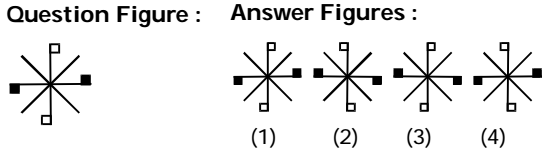1. As per the given above question figure ,
Figure ( 2 ) of the answer figures is exactly the water image of the given original image . Thus , Given image will become opposite in water across the given line.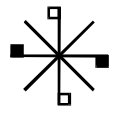##### Correct Option: B

As per the given above question figure ,
Figure ( 2 ) of the answer figures is exactly the water image of the given original image . Thus , Given image will become opposite in water across the given line.So , option B is correct figure .1. Choose the correct water image of the question figure out of the four answer figures given: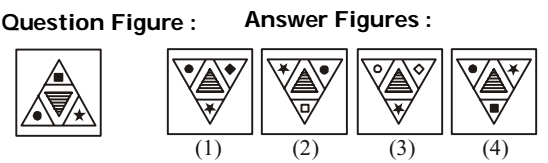1. As we know that Water image is the reflection of an object into the water . It appears by inverting an object vertically i.e. upside down. The water image always be opposite the original image across the given line. It will become opposite to the given line .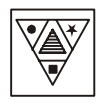##### Correct Option: D

As we know that Water image is the reflection of an object into the water . It appears by inverting an object vertically i.e. upside down. The water image always be opposite the original image across the given line. It will become opposite to the given line .
Therefore , required answer will be option D .1. Which of the answer figures is exactly the water image of the given figure?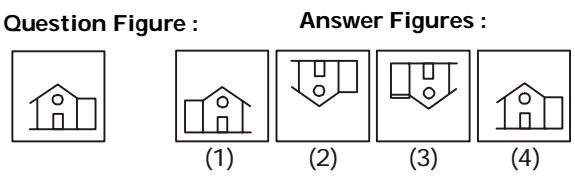1. On the basis of above given question , we can say that
Water image is the reflection of an object into the water . It appears by inverting an object vertically i.e. upside down. The water image always be opposite the original image across the given line. It will become opposite to the given line .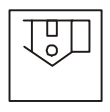##### Correct Option: B

On the basis of above given question , we can say that
Water image is the reflection of an object into the water . It appears by inverting an object vertically i.e. upside down. The water image always be opposite the original image across the given line. It will become opposite to the given line .
Therefore , required answer will be option B .